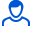Content: teorver_5577.doc (28.00 KB)

Positive responses: 0
Negative responses: 0

Refunds: 05577. x̅=21, n=100, σ=12. Determine the confidence interval for estimating with reliability γ=0.975 the unknown mathematical expectation a of the normally distributed feature X of the general population, if the sample mean x̅в=21, the sample size n=100 and the general standard deviation σ=12 are known.
Detailed solution. Decorated in Microsoft Word 2003 (Quest decided to use the formula editor)
No feedback yet